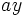# Direct product of D8 and Z2

(diff) ← Older revision | Latest revision (diff) | Newer revision → (diff)
View a complete list of particular groups (this is a very huge list!)[SHOW MORE]

This particular group is a finite group of order: 16

## Definition

This group is defined as the external direct product of the dihedral group of order eight and the cyclic group of order two.

A presentation for it is:$:= \langle a,x,y \mid a^4 = x^2 = y^2 = e, xax = a^{-1}, ya = ay, xy = yx \rangle$.

## Elements

### Upto conjugacy

There are ten conjugacy classes:

1. The identity element. (1)
2. The element$a^2$. (1)
3. The element$y$. (1)
4. The element$a^2y$. (1)
5. The two-element conjugacy class comprising$a$ and$a^3$. (2)
6. The two-element conjugacy class comprising$ay$ and$a^3y$. (2)
7. The two-element conjugacy class comprising$x$ and$a^2x$. (2)
8. The two-element conjugacy class comprising$xy$ and$a^2xy$. (2)
9. The two-element conjugacy class comprising$ax$ and$a^3x$. (2)
10. The two-element conjugacy class comprising$axy$ and$a^3xy$. (2)

### Upto automorphism

Under the action of the automorphism group, the conjugacy classes (3) and (4) are in the same orbit -- in other words,$y$ and$a^2y$ are related by an automorphism. The conjugacy classes (5) and (6) are equivalent, and the conjugacy classes (7)-(10) are equivalent. Thus, the equivalence classes have sizes$1,1,2,4,8$.

## Group properties

Property Satisfied Explanation Comment
Abelian group No$a$ and$x$ don't commute
Nilpotent group Yes Prime power order implies nilpotent
Metacyclic group No
Supersolvable group Yes Finite nilpotent implies supersolvable
Solvable group Yes Nilpotent implies solvable
T-group No$\langle x \rangle \triangleleft \langle a^2,x \rangle$, which is normal, but$\langle x \rangle$ is not normal Smallest example for normality is not transitive.
Monolithic group No Center is Klein four-group, any subgroup of order two is minimal normal.
One-headed group No Distinct maximal subgroups of order eight.
SC-group No
ACIC-group Yes Every automorph-conjugate subgroup is characteristic
Rational group Yes Any two elements that generate the same cyclic group are conjugate Thus, all characters are integer-valued.
Rational-representation group Yes All representations over characteristic zero are realized over the rationals. Contrast with quaternion group, that is rational but not rational-representation.